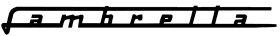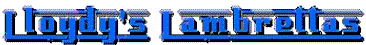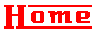Gearbox Ratios & Oil

Gearbox calculations simplified

To calculate the gearbox final drive gear ratio you divide loose gear teeth by cluster teeth.
To calculate the gearbox primary gear ratio (the sprockets) you divide the clutch sprocket by the front sprocket.
To calculate the overall ratio you multiply the two together
To calculate the percentage drop from gear to gear you get the ratio of the gear you are in (IN) and the gear you are changing to (TO). The formula is ((TO-IN)/IN)*100.
Subtract IN from TO then divide it by IN, then multiply it by 100. This will give a negative percent i.e. pacemaker is 19.65% (good), nice. SX 150 is 29%, (not good)

Gear Ratios

Oil

Gearbox exploded diagram

MB Developments gearbox information

Scooterhelp Lambretta gearing calculator

Finding Neutral Hint: Position the selector arm to point to the "X" of the SX engine number or the "I" on the LI casing.